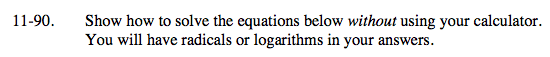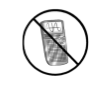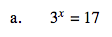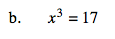### Home > A2C > Chapter Ch11 > Lesson 11.2.4 > Problem11-90

11-90.
1. Show how to solve the equations below without using your calculator. You will have radicals or logarithms in your answers. Homework Help ✎

1. 3x = 17

2. x3 = 17Take the log of both sides.

$\log (3^\textit{x})=\log (17)$

Apply the Power Property of Logarithms.

x · log(3) = log(17)

$\textit{x}= \frac{ \log(17) }{ \log(3)}$Find the cubic root of both sides of the equation.

$\textit{x}= \sqrt{ 17 }$